Next: Least Squares Approaches Up: Statistical Background Previous: Bayes Theorem

## Maximum Likelihood

Starting with Bayes theorem we can extend the joint probability equation to three and more events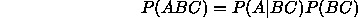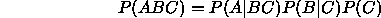For n events with probabilities computed assuming a particular interpretation of the data Y (for example a model)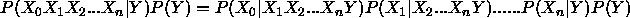Maximum Likelihood statistics involves the identification of the event Y which maximizes such a probability. In the absence of any other information the prior probability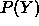is assumed to be constant for all Y . For large numbers of variables this is an impractical method for probability estimation. Even if the events were simple binary variables there are clearly an exponential number of possible values for even the first term in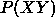requiring a prohibitive amount of data storage. In the case where each observed event is independent of all others we can write.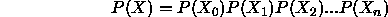Clearly this is a more practical definition of joint probability but the requirement of independence is quite a severe restriction. However, in some cases data can be analysed to remove these correlations, in particular the use of an appropriate data model (such as in least squares fitting) and processes for data orthogonalisation (including principle component analysis). For these reasons all common forms of maximum likelihood definitions assume data independence.

Probability independence is such an important concept it is worth defining carefully. If knowledge of the probability of one variable A allows us to gain knowledge about another event B then these variables are not independent. Put in a way which is easily visualised, if the distribution of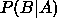over all possible values of B is constant for all A then the two variables are independent. Assumptions of independence of data can be tested graphically by plotting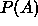against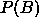or A against B if the variables are directly monotonically related to their probabilities.

Unfortunately, under some circumstances, observable evidence towards a hypothesis cannot be combined into a single measure. Such problems are called Multi Objective problems, under such circumstances it may be impossible to define a unique solution to a problem and a whole set of candidate solution exist for various combinations of trade-off between the two objectives. This is best seen in the area of Genetic Algorithms where the set of optimal solutions lies along what is known as the Pareto Front. Further discussion of these techniques is beyond the scope of this tutorial, except to say that multi-objective optimization should be avoided if a probabilistic combination approach is applicable.Next: Least Squares Approaches Up: Statistical Background Previous: Bayes Theorem

Bob Fisher
Fri Mar 28 14:12:50 GMT 1997